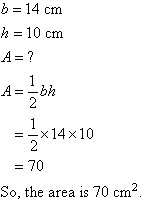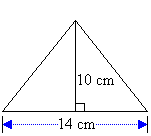Year 9 Interactive Maths - Second Edition

## Area

Area is the size of the region enclosed within a plane figure. To the carpenter or carpet layer, who is concerned with the amount of material to be used, measurement of area is very important.

Area is measured in square units.  The most frequently used units of area are mm2, cm2 and m2.  Land areas are often given in hectares (ha).

1 ha = 10 000 m2

The area of plane figures are often required to solve real world problems.

### Area of a Square

A square with sides of length s units has an area of A square units given by the formula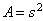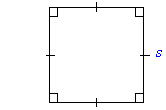#### Example 6

Find the area of a square flower-bed of side 4.5 m.

##### Solution: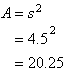So, the area is 20.25 m2.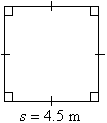###### Note:

To find the area of a region enclosed within a plane figure, draw a diagram and write an appropriate formula.  Then substitute the given values and use a calculator, if necessary, to obtain the required area.

### Area of a Rectangle

A rectangle with length l units and width w units has an area of A square units given by the formula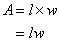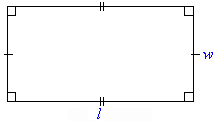#### Example 7

Find the area of a rectangular field 40 m long and 30 m wide.

##### Solution: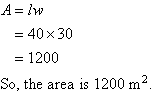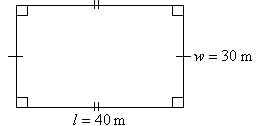### Area of a Triangle

A triangle with base b units and height h units has an area of A square units given by the formula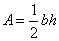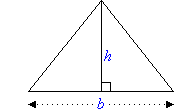#### Example 8

Find the area of a triangle with base 14 cm and height 10 cm.

##### Solution: